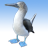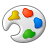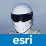# Polyline forming closed segment

1280
6
Jump to solution
10-08-2013 08:21 PMby Anonymous User
Not applicable
Original User: e_saurabh

[ATTACH=CONFIG]28156[/ATTACH][ATTACH=CONFIG]28157[/ATTACH]
Hi,

We are facing a real complex issue regarding creating the Polyline from the coordinate values.

The issue is regarding polyline geometry, when we get the coordinates of the SQL Geometry and trying to create ARCGIS Geometry using PointCollection and Polyline class of IPointCollection and IGeometry interfaces through AddPointCollection method, the last and first vertices of the polyline geometry is getting joined automatically and forms a line segment and creates a shape like polygon and then pushes this to ArcSDE. This happens for simple and complex polylines. Attached is the actual polyline in the SQl Server geometry and the polyline which forms a close segment in ArcSDE GDB. We are using SQl Server 2008, ArcGIS Server 10.0 and ArcSDE 10.0. Attached are the images.

This has become a show stopper for our application. If anyone can please respond quickly on this.

Regards,
Saurabh.
1 Solution

Accepted SolutionsRegular Contributor III
Here you are creating a new polyline:
polyline = (Polyline)serverContext.CreateObject("esriGeometry.Polyline");

Here you are performing a QI from IPoint to IPointCollection:
pointCollection = polyline as IPointCollection;

Here you are adding points to the point collection:
for (int count = 0; count < geoDataArray.Length; count++)
{
pointArray = geoDataArray[count].Split(new char[] { ',' });
latitude = Convert.ToDouble(pointArray.ToString());
longitude = Convert.ToDouble(pointArray.ToString());
point.X = latitude;
point.Y = longitude;
pointCollection.AddPoint(point, ref missingObject, ref missingObject);
}

At this point you have created the polyline and added the points.
But, here you add the points again by adding the point collection to the polyline:
polyline.AddPointCollection(pointCollection);

You don't want to do that because you're adding the points twice.
6 RepliesRegular Contributor II
without the actual code fragment it is very difficult to help.by Anonymous User
Not applicable
Original User: e_saurabh

without the actual code fragment it is very difficult to help.

Please find the code below...

IFeature feature = featureClass.CreateFeature();
IGeometry lineGeometry;
Polyline polyline;
string shapeGeoData = string.Empty;
IPointCollection pointCollection;
shapeGeoData = geoData.Remove(0, 10);
shapeGeoData = shapeGeoData.Replace("(", "");
shapeGeoData = shapeGeoData.Replace(")", "");
shapeGeoData = shapeGeoData.Replace(", ", ",");
shapeGeoData = shapeGeoData.Replace(" ", ";");
shapeGeoData = shapeGeoData.Replace(",", " ");
shapeGeoData = shapeGeoData.Replace(";", ",");
shapeGeoData = shapeGeoData.TrimStart(',');

polyline = (Polyline)serverContext.CreateObject("esriGeometry.Polyline");
pointCollection = polyline as IPointCollection;

string value = shapeGeoData
string[] geoDataArray = value.Split(new char[] { ' ' });
IPoint point = (IPoint)serverContext.CreateObject("esriGeometry.Point");
object missingObject = Type.Missing;

for (int count = 0; count < geoDataArray.Length; count++)
{
pointArray = geoDataArray[count].Split(new char[] { ',' });
latitude = Convert.ToDouble(pointArray.ToString());
longitude = Convert.ToDouble(pointArray.ToString());
point.X = latitude;
point.Y = longitude;
pointCollection.AddPoint(point, ref missingObject, ref missingObject);
}

polyline.AddPointCollection(pointCollection);

ISpatialReferenceFactory spatialReferenceFactory;
ISpatialReferenceResolution spatialReferenceResolution;
ISpatialReferenceTolerance spatialReferenceTolerance;
ISpatialReference spatialReference=null;

SpatialReferenceFactory = serverContext.CreateObject("esriGeometry.SpatialReferenceEnvironment") as ISpatialReferenceFactory;

//Create a projected coordinate system and define its domain, resolution, and x,y tolerance.
spatialReferenceResolution = spatialReferenceFactory.CreateGeographicCoordinateSystem(SRID) as ISpatialReferenceResolution;
spatialReferenceResolution.ConstructFromHorizon();
spatialReferenceTolerance = spatialReferenceResolution as ISpatialReferenceTolerance;
spatialReferenceTolerance.SetDefaultXYTolerance();
spatialReference = spatialReferenceResolution as ISpatialReference;

lineGeometry.SpatialReference = spatialReference

ITopologicalOperator topologicalOperator = polyline as ITopologicalOperator;
topologicalOperator.Simplify();

feature.Shape = lineGeometry;
feature.Store();Regular Contributor III
Here you are creating a new polyline:
polyline = (Polyline)serverContext.CreateObject("esriGeometry.Polyline");

Here you are performing a QI from IPoint to IPointCollection:
pointCollection = polyline as IPointCollection;

Here you are adding points to the point collection:
for (int count = 0; count < geoDataArray.Length; count++)
{
pointArray = geoDataArray[count].Split(new char[] { ',' });
latitude = Convert.ToDouble(pointArray.ToString());
longitude = Convert.ToDouble(pointArray.ToString());
point.X = latitude;
point.Y = longitude;
pointCollection.AddPoint(point, ref missingObject, ref missingObject);
}

At this point you have created the polyline and added the points.
But, here you add the points again by adding the point collection to the polyline:
polyline.AddPointCollection(pointCollection);

You don't want to do that because you're adding the points twice.by Anonymous User
Not applicable
Original User: e_saurabh

Here you are creating a new polyline:
polyline = (Polyline)serverContext.CreateObject("esriGeometry.Polyline");

Here you are performing a QI from IPoint to IPointCollection:
pointCollection = polyline as IPointCollection;

Here you are adding points to the point collection:
for (int count = 0; count < geoDataArray.Length; count++)
{
pointArray = geoDataArray[count].Split(new char[] { ',' });
latitude = Convert.ToDouble(pointArray.ToString());
longitude = Convert.ToDouble(pointArray.ToString());
point.X = latitude;
point.Y = longitude;
pointCollection.AddPoint(point, ref missingObject, ref missingObject);
}

At this point you have created the polyline and added the points.
But, here you add the points again by adding the point collection to the polyline:
polyline.AddPointCollection(pointCollection);

You don't want to do that because you're adding the points twice.

Hi Neil,

Thanks a lot!!  Your click worked right away. Now this works fine. Probably some coding mistakes.

Regards,
Saurabh.byEsri Regular Contributor
You may also want to check or change:

`point.X = latitude;point.Y = longitude;`by Anonymous User
Not applicable
Original User: e_saurabh

Sure Sean.. will follow up a check on this. Thanks.

Regards,
Saurabh.